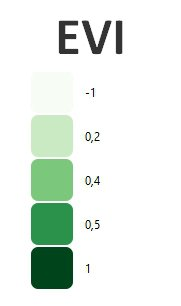What is Enhanced Vegetation Index (EVI)?

The Enhanced Vegetation Index (EVI) is an advanced vegetation index created with higher sensitivity to biomass, atmospheric background, and soil condition. It is regarded as the modified version of Normalized Difference Vegetation Index (NDVI) with a high potentiality of vegetation monitoring by correcting all the external noises. Vegetation Indices are calculated from the bilateral surface reflectance that has been concealed for cloud, smoke, aerosols, water, cloud shadows, etc.

The value of EVI ranges from 0 to 1. A value closer to 1 represents healthy vegetation and a value closer to 0 represents unhealthy vegetation. In recent times, EVI has proved to be an efficient technique in vegetation change detection and derivation of canopy biophysical characteristics of a particular region. Bright features such as clouds and white buildings, along with dark features such as water, can result in anomalous pixel values in an EVI image.

How do you calculate EVI?

EVI uses the blue, red, and NIR band. It incorporates an “L” value to adjust for canopy background, “C” values as coefficients for atmospheric resistance and values from the blue band (B). These enhancements allow for index calculation as a ratio between the R and NIR values, while reducing the background noise, atmospheric noise, and saturation in most cases.

EVI is calculated as follow:

EVI = G * ((NIR - R) / (NIR + C1 * R – C2 * B + L)
EVI (Sentinel 2) = 2.5 * ((B8 – B4) / (B8 + 6 * B4 – 7.5 * B2 + 1)).
EVI (Landsat 8) = 2.5 * ((B5 – B4) / (B5 + 6 * B4 – 7.5 * B2 + 1)).
EVI (Landsat 4-7) = 2.5 * ((B4 – B3) / (B4 + 6 * B3 – 7.5 * B1 + 1)).

Application of EVI, Rostenice farm